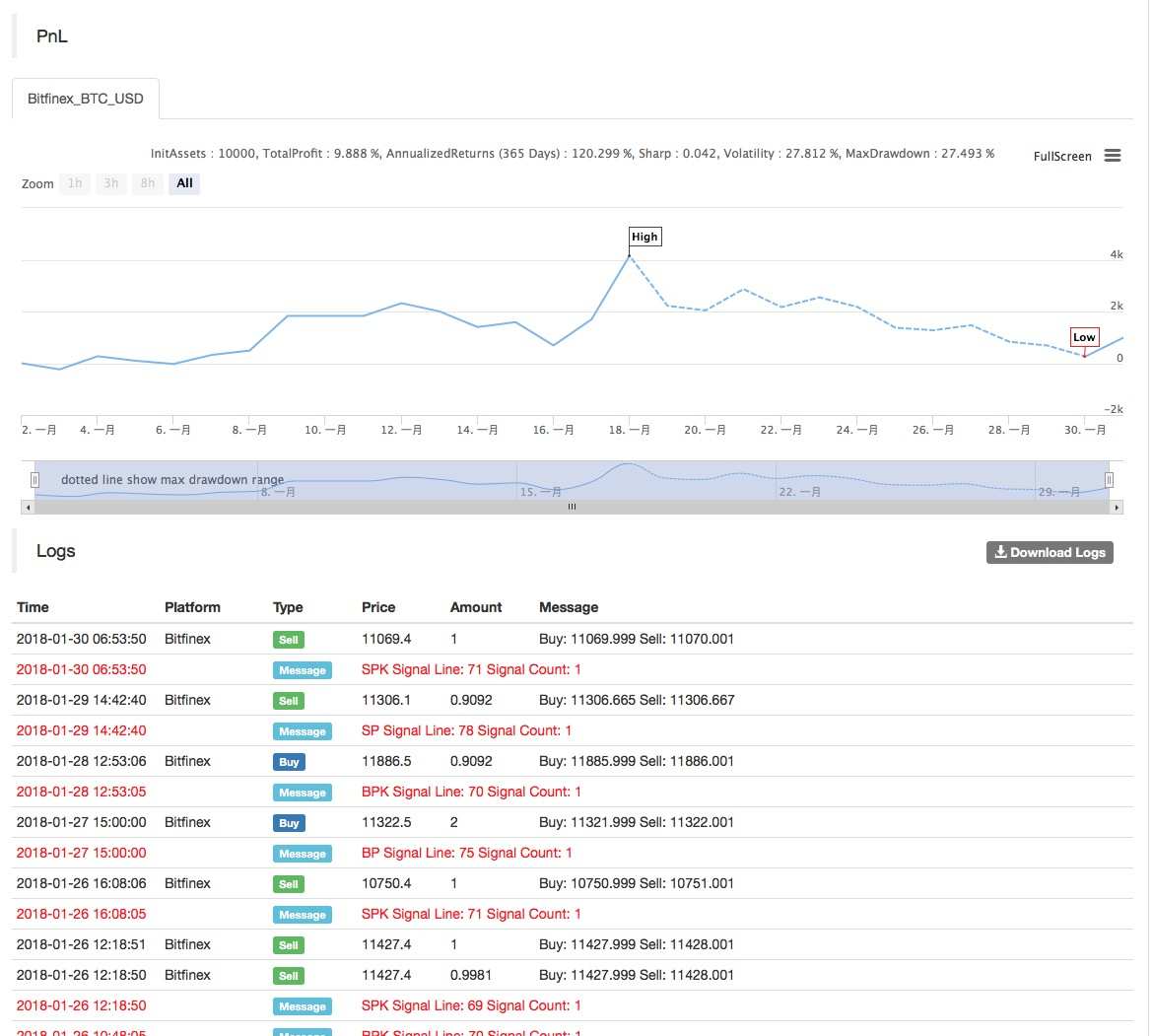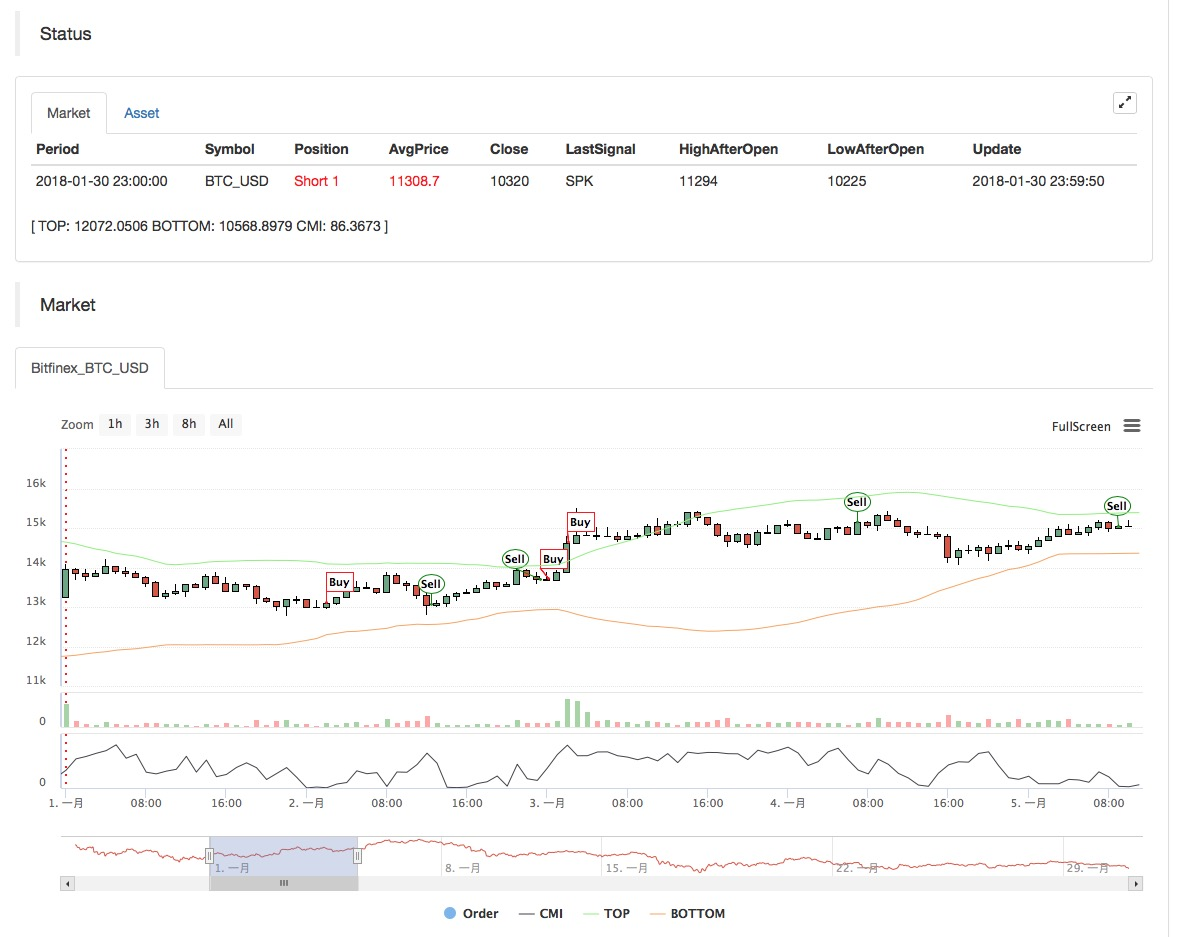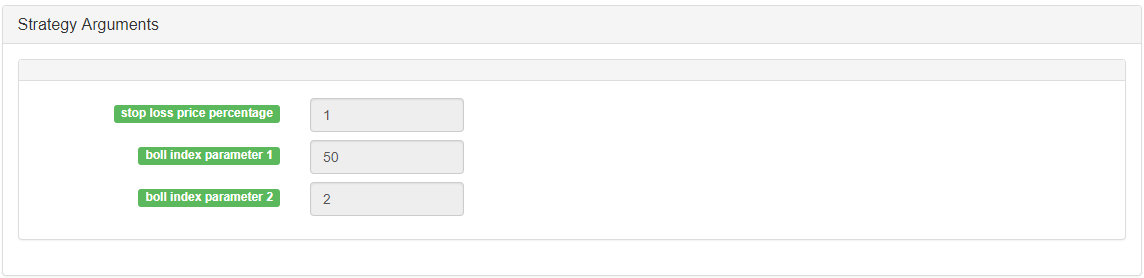# [原创] 恒温器策略在发明者量化平台的实践与应用

Abs（收盘价 - 收盘价）/（最高价（30）- 最低价（低价，30日）* 100

• 主图：
上轨公式：TOP^^MAC+N_TMPTMP;//布林通道上轨
下轨公式：BOTTOM^^MAC-N_TMPTMP;//布林通道下轨

• 副图：
CMI公式：CMI:ABS(C-REF(C,N_CMI-1))/(HHV(H,N_CMI)-LLV(L,N_CMI))*100;//0-100 取值越大，说明趋势越强，CMI<20震荡模式，CMI>20为趋势

• 代码（My语言）：

``````
MAC:=MA(CLOSE,N);
TMP:=STD(CLOSE,N);
TOP^^MAC+N_TMP*TMP;      // 布林通道上轨
BOTTOM^^MAC-N_TMP*TMP;   // 布林通道下轨
BBOLL:=C>MAC;
SBOLL:=C<MAC;
N_CMI:=30;

CMI:ABS(C-REF(C,N_CMI-1))/(HHV(H,N_CMI)-LLV(L,N_CMI))*100; //0-100 取值越大，说明趋势越强，CMI<20震荡模式，CMI>20为趋势

N_KD:=9;
M1:=3;
M2:=3;
RSV:=(CLOSE-LLV(LOW,N_KD))/(HHV(HIGH,N_KD)-LLV(LOW,N_KD))*100; //收盘价与N周期最低值做差，N周期最高值与N周期最低值做差，两差之间做比值。

K:=SMA(RSV,M1,1); //RSV的移动平均值
D:=SMA(K,M2,1);   //K的移动平均值
MIND:=30;
BKD:=K>D AND D<MIND;
SKD:=K<D AND D>100-MIND;

// 震荡模式
BUYPK1:=CMI < 20 AND BKD;  //震荡多单买平开
SELLPK1:=CMI < 20 AND SKD; //震荡空单卖平开

// 趋势模式下原有震荡持仓的处理
SELLY1:=REF(CMI,BARSBK) < 20 AND C>BKPRICE*(1+0.01*STOPLOSS*3) AND K<D; //震荡多单止盈

// 趋势模式
BUYPK2:=CMI >= 20 AND C > TOP;        // 趋势多单买平开
SELLPK2:=CMI >= 20 AND C < BOTTOM;    // 趋势空单卖平开

// 趋势模式下原有震荡持仓的处理
SELLY2:=REF(CMI,BARSBK) >= 20 AND C>BKPRICE*(1+0.01*STOPLOSS*3) AND SBOLL;//趋势多单止盈
SELLS2:=REF(CMI,BARSBK) >= 20 AND C<BKPRICE*(1-0.01*STOPLOSS) AND SBOLL;//趋势多单止损

IF BARPOS>N THEN BEGIN
SELLPK1,SPK;
SELLPK2,SPK;
END
SELLY1,SP(BKVOL);
SELLY2,SP(BKVOL);
SELLS2,SP(BKVOL);
``````